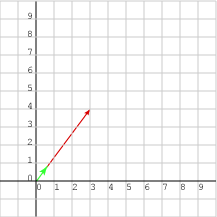Normalize (3, 4)T.

The vector is the hypotenuse of a 3-4-5 right triangle, so its length is 5. The unit vector is:

``` 1/5 × (3,4)T  =  (3/5, 4/5)T  =  (0.6, 0.8)T
```

# Direction of a Unit VectorScaling changes the length of a vector but not its direction. If vu is the unit vector corresponding to v, then vu and v have the same orientation.

This sounds plausible, but a demonstration might not hurt:

```Start with v = (3, 4)T as above.

Form vu = (3/5, 4/5)T.

The direction of v is arc tan( 4/3 ).

The direction of vu is
arc tan( (4/5) / (3/5) ) =
arc tan( (4/5) × (5/3) ) =
arc tan( 4/3 )
```

### QUESTION 11:

Construct a unit vector in the same direction as w = (4, 6)T.